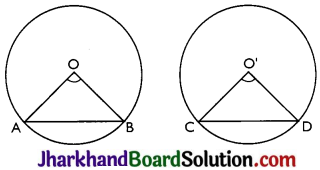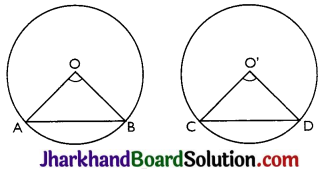# JAC Class 9 Maths Solutions Chapter 10 Circles Ex 10.2

Jharkhand Board JAC Class 9 Maths Solutions Chapter 10 Circles Ex 10.2 Textbook Exercise Questions and Answers.

## JAC Board Class 9th Maths Solutions Chapter 10 Circles Ex 10.2

Page-173

Question 1.
Recall that two circles are congruent if they have the same radii. Prove that equal chords of congruent circles subtend equal angles at their centres.
Given: AB = CD (Equal chords)
To prove: ∠AOB = ∠CO’D
Proof: In AAOB and ACO’D,OA = O’C
OB = O’D
AB = CD
∴ ∆AOB ∆CO’D
(SSS congruence criterion)
Thus, ∠AOB ≅ ∠CO’D by CPCT.
i.e. Equal chords of congruent circles subtend equal angles at their centres.Question 2.
Prove that if chords of congruent circles subtend equal angles at their centres, then the chords are equal.